UIHistories Project: A History of the University of Illinois by Kalev Leetaru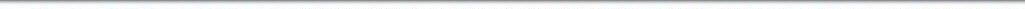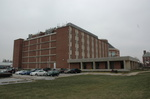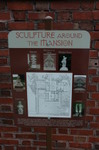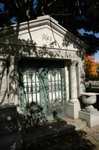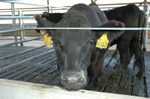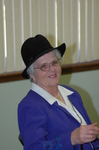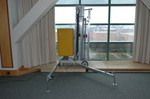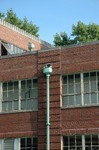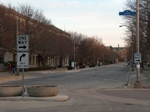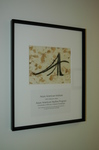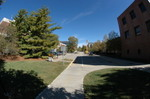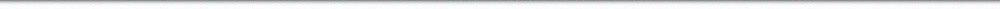N A V I G A T I O N D I G I T A L L I B R A R Y

## Repository: UIHistories Project: Book - Century of Physics (1973) [PAGE 30]

Caption: Book - Century of Physics (1973)
This is a reduced-resolution page image for fast online browsing.

< Previous Page [Displaying Page 30 of 39] Next Page >
[VIEW ALL PAGE THUMBNAILS]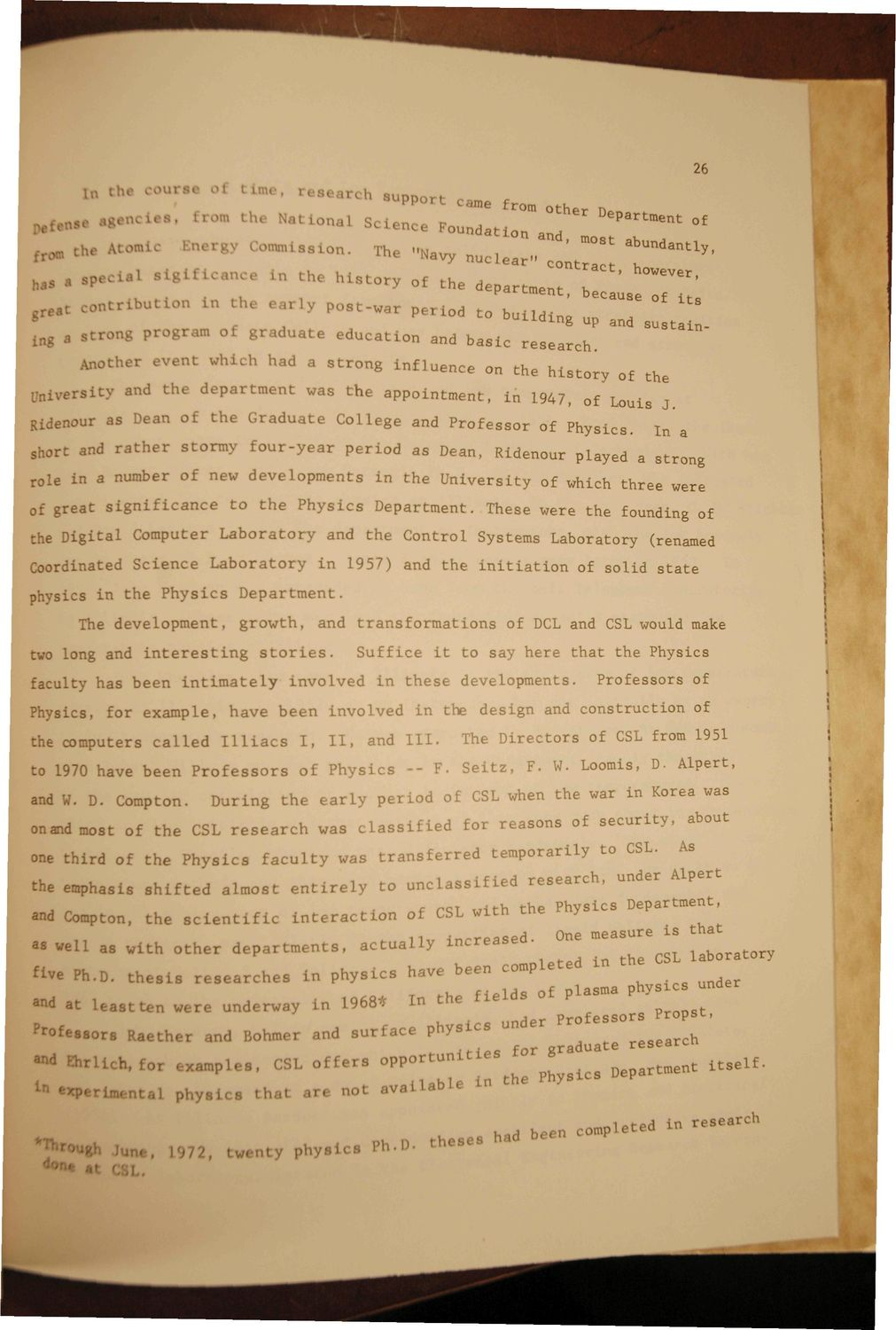### EXTRACTED TEXT FROM PAGE:

26

11 se

* ° f agents, *• •'

C

U

M tI

' " ' " a the National

l n:i1

earch

s u p p o r t came fro Sctenc * < ^ t ^ ^ The "Navy n u c l e a r "

the

DePartment

°

f

*n tl

has

TO —

amission.

' ^

abUndantly h0VeV6r

'

a « «

i n t h e h i s t o r y of

departme^T^' research.

'

ng

a s t r o n g program oi g r a d u a t e e d u c a t i o n and b a s i c

Another e v e n t which had a s t r o n g i n f l u e n c e on t h e h i s t o r y of the n i v e r s i t v and t h e d e p a r t m e n t was t h e a p p o i n t m e n t , i n 1947, of Louis J In a In a .... strong

Ridenour as Dean of t h e G r a d u a t e CoLlege and P r o f e s s o r of P h y s i c s . -~- — ~ ^ o o ^ ui. r n y s i c s . chort and r a t h e r s t o r m y f o u r - y e a r p e r i o d as Dean. Ridpnnnt- «i « , ^ a p e r i o d as Dean, Ridenour played „ ore and r a t h e r s t o r m y f o u r - y e a r

rol e in a number of new d e v e l o p m e n t s m t h e U n i v e r s i t y of which t h r e e were tu of g r e a t s i g n i f i c a n c e t o t h e P h y s i c s D e p a r t m e n t . These were t h e founding of

the D i g i t a l Computer L a b o r a t o r y and t h e C o n t r o l Systems Laboratory (renamed Coordinated S c i e n c e L a b o r a t o r y i n 1957) and t h e i n i t i a t i o n of s o l i d s t a t e physics in t h e P h y s i c s The d e v e l o p m e n t , Department. growth, and t r a n s f o r m a t i o n s of DCL and CSL would make Suffice i t t o say h e r e t h a t t h e Physics P r o f e s s o r s of

two long and i n t e r e s t i n g s t o r i e s . faculty has b e e n i n t i m a t e l y

involved in t h e s e developments.

Physics, for e x a m p l e , h a v e b e e n i n v o l v e d i n the d e s i g n and c o n s t r u c t i o n of the computers c a l l e d I l l i a c s to 1970 have b e e n P r o f e s s o r s and W. D. Compton. I, II, and 1 1 1 . The D i r e c t o r s of CSL from 1951 F. W. Loomis, D. A l p e r t ,

of P h y s i c s - - F. S e i t z ,

D u r i n g t h e e a r l y p e r i o d of CSL when t h e war in Korea was for r e a s o n s of s e c u r i t y , t e m p o r a r i l y to CSL. about As

onandmost of t h e CSL r e s e a r c h was c l a s s i f i e d one t h i r d of t h e P h y s i c s the emphasis s h i f t e d

f a c u l t y was t r a n s f e r r e d

t o u n c l a s s i f i e d r e s e a r c h , under Alpert f rciT w i t h t h e P h y s i c s Department, and Compton, t h e s c i e n t i f i c i n t e r a c t i o n of CSL w i t n y A Onp niGcisurG i s t h a t as well as w i t h o t h e r d e p a r t m e n t s , a c t u a l l y i n c r e a s e . ^ laboratory ^ v e Ph.D. t h e s i s r e s e a r c h e s i n p h y s i c s h a v e b e e n completed r^ T f l , o f i e l d s of plasma p h y s i c s under and at l e a s t ten w e r e u n d e r w a y Ln 1968* In t h e f i e l d s Aar P r o f e s s o r s r r o p s u , Professors R a e t h e r and Bohmer and s u r f a c e p h y s i c s under _earch - o r . K a e t h e r and Bonmer ana • — * research duate and » r l i c h , f o r i r m p l e s , CSL o f f e r s o p p o r t u n i t y ^ ^ ^ i t elfDepartment

almost e n t i r e l y

In

perimental

o h v a i c s t h a t a r e not

available

ompleted in research *h

*

ne, ) /2, twenty physics Ph.D. theses had been c